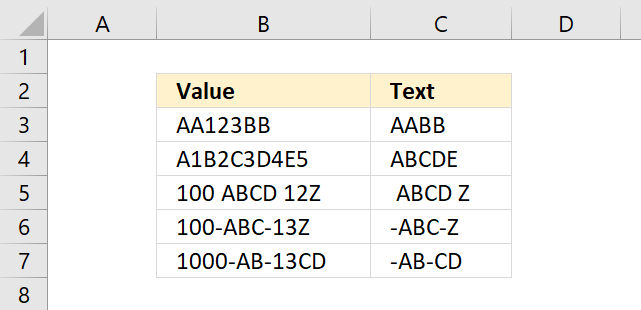Author: Oscar Cronquist Article last updated on November 15, 2018The array formula in cell C3:C7 extracts everything except numbers from cell B3.

The following formula contains the TEXTJOIN function and it works only in Excel 2016.

=TEXTJOIN("", TRUE, TEXT(MID(B3, ROW(\$A\$1:INDEX(\$A\$1:\$A\$1000, LEN(B3))), 1), ""))

It allows you to filter values up to 1000 characters and you can easily change that limit by changing this cell reference: \$A\$1:\$A\$1000 in the formula above.

### Explaining the array formula in cell C3

The following part builds a cell reference that has as many values as there are characters in cell B3.

\$A\$1:INDEX(\$A\$1:\$A\$1000, LEN(B3))

becomes

\$A\$1:INDEX(\$A\$1:\$A\$1000, 7) and returns \$A\$1:\$A\$7.

The ROW function then converts the cell range to an array containing the numbers 1, 2, 3 ... up to the number of characters in cell B3.

ROW(\$A\$1:INDEX(\$A\$1:\$A\$1000, LEN(B3)))

becomes

ROW(\$A\$1:\$A\$7) and returns this array: {1, 2, 3, 4, 5, 6, 7}.

The MID function then splits the values into an array of 7 values containing a character each.

MID(B3, ROW(\$A\$1:INDEX(\$A\$1:\$A\$1000, LEN(B3))), 1)

becomes

MID(B3, {1, 2, 3, 4, 5, 6, 7}, 1) and returns {"A";"A";"1";"2";"3";"B";"B"}.

The TEXT function removes the numbers from the array.

TEXT(MID(B3, ROW(\$A\$1:INDEX(\$A\$1:\$A\$1000, LEN(B3))), 1), "")

becomes

TEXT({"A";"A";"1";"2";"3";"B";"B"}, "") and returns {"A";"A";"";"";"";"B";"B"}.

Lastly, the TEXTJOIN function concatenates all values in the array without a delimiting character, empty values are ignored.

TEXTJOIN("", TRUE, TEXT(MID(B3, ROW(\$A\$1:INDEX(\$A\$1:\$A\$1000, LEN(B3))), 1), ""))

becomes

TEXTJOIN("", TRUE, {"A";"A";"";"";"";"B";"B"}) and returns AABB in cell C3.